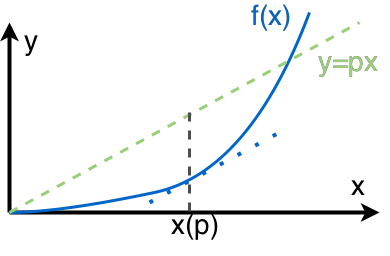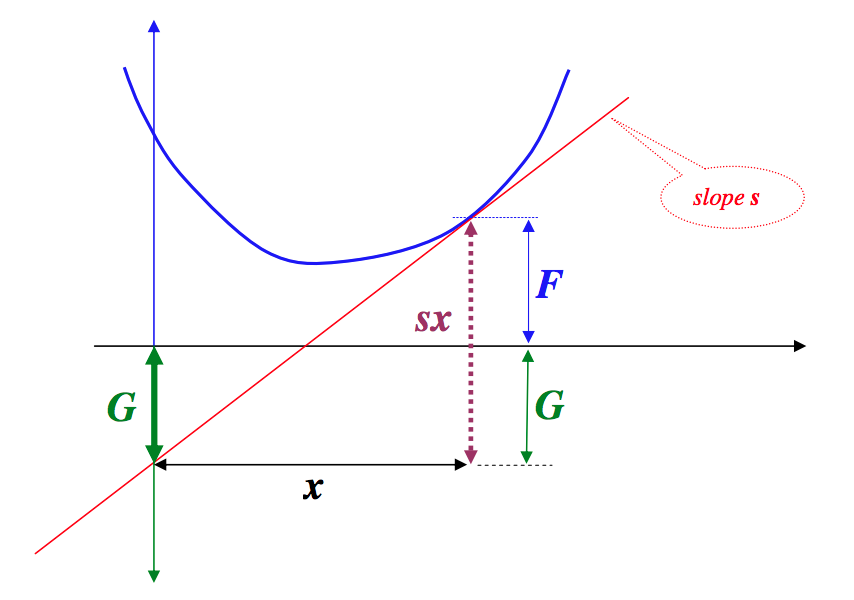# Useful Math Tricks¶

## Functional Derivative¶

By definition,  functional derivative of a functional $$G[f]$$ with respect to $$f$$ along the ‘direction’ of $$h$$ is

$\delta G[f][h] = \frac{d}{d\epsilon} G[f+\epsilon h]\vert_{\epsilon=0}.$

As an example, the functional derivative of $$G[f]=\int dx f^n(x)$$ $$\delta G[f][h]$$ is

$\begin{split}\delta G[f][h] &= \frac{d}{d\epsilon} G[f+\epsilon h] \vert_{\epsilon=0} \\ & = \int nf^{n-1}(x) h(x) dx.\end{split}$

Now the problem appears. We have an unknown function $$h$$ which makes sense because we haven’t specify a direction of the derivative yet.

For a physicist, the savior of integral is Dirac delta. So we use delta distribution as the direction in the functional derivative of action which is an integral,

$\frac{\delta G[f]}{\delta f(y)} = \delta G[f][\delta_y].$

It can be ambiguous to just write down $$\delta_y$$ without an example. Here is the previous example continued,

$\begin{split}\frac{\delta G[f(y)]}{\delta f(y)} &= \int nf^{n-1}(x) h(x) dx \vert_{h(x)= \delta(x-y)} \\ & = \int nf^{n-1}(x) \delta(x-y) dx \\ & = n f^{n-1}(y) .\end{split}$

It seems that we can just think of $$f$$ as a variable then take the ordinary derivative with respect to it. It is NOT true.

Consider such a functional $$G[f]=\int (f'(x))^2 dx$$ where ‘ means the derivative of $$f(x)$$.

$\begin{split}\frac{\delta G[f]}{\delta f} & = -\int dx 2 f''(x) h(x) \vert_{h(x)=\delta(x-y)} \\ & = -2 f''(y) ,\end{split}$

which is not that straightforward to understand from function derivatives.

  Chapter 15 of Physical Mathematics

## Legendre Transformation¶

Legendre transformation is NOT just some algebra. Given $$f(x)$$ as a function of $$x$$, which is shown in blue, we could find the distance between a line $$y=px_i$$ and the function value $$f(x_i)$$.Fig. 2 Meaning of Legendre transformation

However, as we didn’t fix $$x$$, this means that the distance

$F(p,x) = p x - f(x)$

varies according to $$x$$. This is a transformation that maps a function $$f(x)$$ to some other function $$F(p,x)$$ which depends on the parameter $$p$$. A more pedagogical way of writing this is

$px = F(x,p) + f(x).$

To have a Legendre transformation, let’s choose a relation between $$x$$ and $$p$$. One choice is to make sure we have a maximum distance given $$p$$, which means the $$x$$ we choose is the point that makes the slope of $$f(x)$$ the same as the line $$y=px$$. In the language of math, the condition we require is

$0 = \frac{\partial F(p,x)}{\partial x} \equiv f'(x) - p,$

which indeed shows that the slope of function and slope of the straight line match eath other at the specified point. Thus we have a relation between $$x$$ and $$p$$.

Substitute $$x(p)$$ back into $$F(p,x)$$, we will get the Legendre transformation $$F(p,x(p))$$ of $$f(x)$$.

Back to the math we learned in undergrad study. A Legendre transformation transforms a function of $$x$$ to another function with variable $$\frac{f(x)}{x}$$. Using $$f(x)$$ and its Legendre transformation $$F(p = p x - f(x(p))$$ as an example, we can show that the slope of $$F(p)$$ is $$x$$,

$\frac{d F(p)}{d p} = x,$

which is intriging because the slope of $$f(x)$$ is $$p$$ in our requirement. We removed the dependence of $$x$$ in $$F(p)$$ because we have this extra constrain.

Let’s Move to Another Level

We require the function $$f(x)$$ is convex (second order derivative is not negative ). This is required because otherwise we would NOT have a one on one mapping of $$x$$ and $$p$$.Fig. 3 This graph shows the Legendre transformation and triangles in which G is actually the F we used before and F in the graph corresponds to f.

One imediately notices the symmety of Legendre transformation on interchanging of F and f.

This graph is taken from this paper Making Sense of the Legendre Transform .

This is the triangle that represents the Legendre transformation.

If we have a slope that vanishes, which means $$f(x)$$ is at minimium, then we have the relation

## Vector Analysis¶

The ultimate trick is to use component form.

$\begin{split}&\vec a \times (\vec b \times \vec c) \\ = & \hat e_i \epsilon_{ijk} a_j (\epsilon_{kmn} b_m c_n ) \\ = & \hat e_i \epsilon_{kij}\epsilon_{kmn} a_j b_m c_n \\ = & \hat e_i ( \delta_{im}\delta_{jn} - \delta_{in}\delta_{jm} )a_j b_m c_n \\ = & \hat e_i \delta_{im}\delta_{jn} a_j b_m c_n - \hat e_i \delta_{in}\delta_{jm} a_j b_m c_n \\ = & \hat e_i a_j b_i c_j - \hat e_i a_j b_j c_i \\ = & \vec b (\vec a\cdot \vec c) - \vec c (\vec a \cdot \vec b) .\end{split}$

One should be able to find the component forms of gradient $$\vec \nabla \cdot$$, divergence $$\vec \nabla \times$$, Laplace operator, in spherical coordinates, cylindrical coordinates and cartisian coordinates.